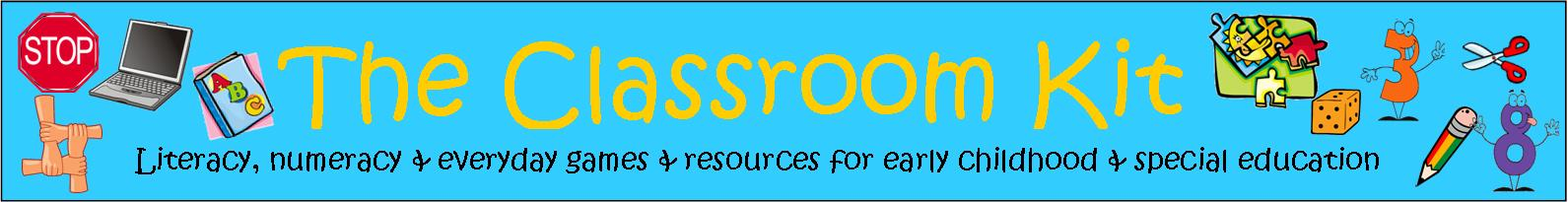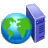## Number Worksheets and Activity Cards: Recognising Numerals and CountingNumber worksheets compliment the hands-on activities that are offered in early childhood classes. Young learners need constant opportunities to manipulate the numerals from 0 to 9, and to practise counting small groups of objects with growing accuracy. Worksheets provide teachers with hard copies of students' efforts, and are useful for assessment and reporting purposes, as evidence of understandings. It is important to include many opportunities for practising these skills within the learning program, and to build in real purposes for counting.

Activity cards support opportunities for students to count larger groups (from 11 to 20, from 20 to 50, and from 20 to 100). Teachers need to ensure that all materials necessary are available when using these cards. Once students are familiar with the procedure of each set of cards, they can work independently within small groups. This allows for different groups of students to work at their own levels of understanding within the class.

## Emergent Worksheets:

 Draw and Count Balloons1-10 Draw and Count Bats1-10 Draw and Count Bugs1-10 Draw and Count Cats1-10 Draw and Count Flowers1-10 Draw and Count Hats1-10 Draw and Count Icecreams1-10 Draw and Count Moons1-10 Draw and Count Pigs1-10 Draw and Count Shells1-10 Draw and Count Snowmen1-10 Draw and Count Teddies1-10 Draw and Count Umbrellas1-10 Draw and Count Worms1-10 Draw and Count Ants1-10 The Ants Go Marching 1-10 Five Little Monkeys Ten Grey Elephants Rocket Race Game Ten Teddies on the Shelf Free resource Match the Kites (numerals 1 to 10) Free resource

Counting Groups to 10: Count each group and write the total in each box.

 Counting Groups to 10 Worksheet 1 Free resource Counting Groups to 10 Worksheet 2 Counting Groups to 10 Worksheet 3 Counting Groups to 10 Worksheet 4 Counting Groups to 10 Worksheet 5 Counting Groups to 10 Worksheet 6 Counting Groups to 10 Worksheet 7 Counting Groups to 10 Worksheet 8 Counting Groups to 10 Worksheet 9 Counting Groups to 10 Worksheet 10

### Adding small groups (up to 10 objects):

Count each group and add them together. Write the sum with the total.

 Adding Groups to 10 Worksheet 1 Adding Groups to 10 Worksheet 2 Adding Groups to 10 Worksheet 3 Adding Groups to 10 Worksheet 4 Adding Groups to 10 Worksheet 5 Adding Groups to 10 Worksheet 6

### Developing the concepts of More and Less with groups from 2 to 10:

Adding 1, 2, and 3 more (with pictures) and introduction of simple algorithm (3+2=5)

What is 1,2, and 3 less (than objects shown) and introduction of simple algorithm (5-2=3)

 Adding more: worksheet 1 Adding more: worksheet 2 Adding more: worksheet 3 Adding more: worksheet 4 Adding more: worksheet 5 Adding more: worksheet 6 What is less: worksheet 1 Free resource What is less: worksheet 2 What is less: worksheet 3 What is less: worksheet 4 What is less: worksheet 5 What is less: worksheet 6

## Perceptual Worksheets:

### Counting groups of objects from 11 to 20:

These worksheets include:

*seeing 10 as a counting unit, with 10 objects grouped together to make counting easier and more efficient

*reading and writing numbers from 11-20

 Counting groups 10-20 Worksheet 1 Counting groups 10-20 Worksheet 2 Counting groups 10-20 Worksheet 3 Counting groups 10-20 Worksheet 4 Counting groups 10-20 Worksheet 5 Counting groups 10-20 Worksheet 6 Counting groups 10-20 Worksheet 7 Counting groups 10-20 Worksheet 8 Counting groups 10-20 Worksheet 9 Counting groups 10-20 Worksheet 10

### The Concept of 'Adding More' With Numbers from 11 to 20:

These worksheets include:

*adding 1, 2 or 3 more to each group

*introducing 'counting on' as a strategy to find 'more'

*writing the algorithm to represent the calculation (15+1=16)

*supporting young learners to develop 'counting on' by providing the objects to count in order to check calculations

 Add 1 More (10-20) Worksheet 1 Add 1 More (10-20) Worksheet 2 Add 1 More (10-20) Worksheet 3 Add 1 More (10-20) Worksheet 4 Add 1 More (10-20) Worksheet 5 Add 1 More (10-20) Worksheet 6 Add 1 More (10-20) Worksheet 7 Add 1 More (10-20) Worksheet 8 Add 1 More (10-20) Worksheet 9 Add 1 More (10-20) Worksheet 10

 Add 2 More (10-20) Worksheet 1 Free resource Add 2 More (10-20) Worksheet 2 Add 2 More (10-20) Worksheet 3 Add 2 More (10-20) Worksheet 4 Add 2 More (10-20) Worksheet 5 Add 2 More (10-20) Worksheet 6 Add 2 More (10-20) Worksheet 7 Add 2 More (10-20) Worksheet 8 Add 2 More (10-20) Worksheet 9 Add 2 More (10-20) Worksheet 10

 Add 3 More (10-20) Worksheet 1 Add 3 More (10-20) Worksheet 2 Add 3 More (10-20) Worksheet 3 Add 3 More (10-20) Worksheet 4 Add 3 More (10-20) Worksheet 5 Add 3 More (10-20) Worksheet 6 Add 3 More (10-20) Worksheet 7 Add 3 More (10-20) Worksheet 8 Add 3 More (10-20) Worksheet 9 Add 3 More (10-20) Worksheet 10

### The Concept of 'Finding Less' With Numbers from 11 to 20:

These worksheets include:

*taking 1, 2 or 3 from each group

*introducing 'counting back' as a strategy to find 'less'

*writing the algorithm to represent the calculation (15-1=14)

*supporting young learners to develop 'counting back' by providing the objects to count in order to check calculations

 What is 1 Less? Worksheet 1 Free resource What is 1 Less? Worksheet 2 What is 1 Less? Worksheet 3 What is 1 Less? Worksheet 4 What is 1 Less? Worksheet 5 What is 1 Less? Worksheet 6 What is 1 Less? Worksheet 7 What is 1 Less? Worksheet 8 What is 1 Less? Worksheet 9 What is 1 Less? Worksheet 10

 What is 2 Less? Worksheet 1 What is 2 Less? Worksheet 2 What is 2 Less? Worksheet 3 What is 2 Less? Worksheet 4 What is 2 Less? Worksheet 5 What is 2 Less? Worksheet 6 What is 2 Less? Worksheet 7 What is 2 Less? Worksheet 8 What is 2 Less? Worksheet 9 What is 2 Less? Worksheet 10

 Missing Number Worms:Worksheet 1 Free resource Missing Number Worms:Worksheet 2 Missing Number Worms:Worksheet 3 Missing Number Worms:Worksheet 4 Missing Number Worms:Worksheet 5 Missing Number Worms:Worksheet 6 Missing Number Worms:Worksheet 7 Missing Number Worms:Worksheet 8 Missing Number Worms:Worksheet 9 Missing Number Worms:Worksheet 10 Before-between-after Worksheet1 Before-between-after Worksheet2 Before-between-after Worksheet3 Before-between-after Worksheet4 Adding Dice to Make 9 Put 5 starfish in 2 rockpools Adding 2 pots of gold Make this number worksheet A Cut into 3 mini-worksheets Make this number worksheet B Cut into 3 mini-worksheets Make this number worksheet C Cut into 3 mini-worksheets Collect 10 counters:ABC (cut into min-worksheets of addition sums) Collect 10 counters:DEF (cut into min-worksheets of addition sums) Collect 10 counters:GHI (cut into min-worksheets of addition sums) Collect 10 counters:KLM (cut into min-worksheets of addition sums)

Weekly Worksheets to Develop Mental Maths Skills at the Perceptual Level:

 Mental Maths Weekly Worksheet P1 Free resource Mental Maths Weekly Worksheet P2 Mental Maths Weekly Worksheet P3 Mental Maths Weekly Worksheet P4 Mental Maths Weekly Worksheet P5 Mental Maths Weekly Worksheet P6 Mental Maths Weekly Worksheet P7 Mental Maths Weekly Worksheet P8 Mental Maths Weekly Worksheet P9 Mental Maths Weekly Worksheet P10

## Figurative Worksheets:

### Counting groups from 10-99

These worksheets include:

*pictorial representations of 2 digit numbers

*groups set out in lines of 10 (or arrays), to make counting easier and to encourage the strategy of counting by 10s when working with 2-digit numbers

*the numerals: 10 20 30 40 50 60 70 80 90 100 at the bottom of each page to support learners at this stage

 Counting groups of 10: Worksheet 1 Free resource Counting groups of 10: Worksheet 2 Counting groups of 10: Worksheet 3 Counting groups of 10: Worksheet 4 Counting groups of 10: Worksheet 5 Counting groups of 10: Worksheet 6 Counting groups of 10: Worksheet 7 Counting groups of 10: Worksheet 8 Counting groups of 10: Worksheet 9 Counting groups of 10: Worksheet 10

 Groups of 3 Free resource

Weekly Worksheets to Promote Mental Maths Skills at the Figurative Level:

 Mental Maths Weekly Worksheet F1 Free resource Mental Maths Weekly Worksheet F2 Mental Maths Weekly Worksheet F3 Mental Maths Weekly Worksheet F4 Mental Maths Weekly Worksheet F5 Mental Maths Weekly Worksheet F6 Mental Maths Weekly Worksheet F7 Mental Maths Weekly Worksheet F8 Mental Maths Weekly Worksheet F9 Mental Maths Weekly Worksheet F10

## Counting On Worksheets:

These worksheets include:

*pictorial representations of 2 digit numbers

*groups set out in lines of 10 (or arrays), to make counting easier and to encourage the strategy of counting by 10s when working with 2-digit numbers

*an introduction to the algorithm for adding two 2-digit numbers vertically (supported by pictorial representation of each numeral)

 Add groups of 10: Worksheet 1 Add groups of 10: Worksheet 2 Add groups of 10: Worksheet 3 Add groups of 10: Worksheet 4 Add groups of 10: Worksheet 5 Add groups of 10: Worksheet 6 Add groups of 10: Worksheet 7 Add groups of 10: Worksheet 8 Add groups of 10: Worksheet 9 Add groups of 10: Worksheet 10 Free resource

### Subtracting groups of 10

These worksheets include:

*pictorial representations of 2 digit numbers

*groups set out in lines of 10 (or arrays), to make counting easier and to encourage the strategy of counting by 10s when working with 2-digit numbers

*an introduction to the algorithm for subtracting two 2-digit numbers vertically (supported by pictorial representation of each numeral)

 Subtract groups of 10: Worksheet 1 Subtract groups of 10: Worksheet 2 Subtract groups of 10: Worksheet 3 Subtract groups of 10: Worksheet 4 Subtract groups of 10: Worksheet 5 Subtract groups of 10: Worksheet 6 Subtract groups of 10: Worksheet 7 Subtract groups of 10: Worksheet 8 Subtract groups of 10: Worksheet 9 Subtract groups of 10: Worksheet 10

## Facile Worksheets: